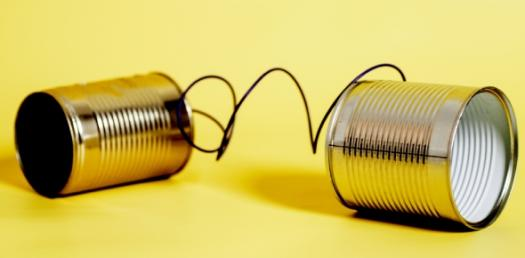# Electronics & Communication Quiz - 2

113 Questions | Attempts: 530
ShareSettingsThis is a very elementary Quiz containing questions from Solid State Devices, Basic Electronics, etc

• 1.
What is Cathode Ray?
• A.

Alpha-Ray

• B.

Beta-ray

• C.

Gamma-ray

• D.

None

• 2.
Who discovered Electron?
• A.

Thomas Alva Edison

• B.

• C.

Alexander G. Bell

• D.

J. J. Thomson

• 3.
What is the polarity of Anode
• A.

Negative

• B.

Positive

• C.

Neutral

• D.

Not applicable

• 4.
What is the polarity of Cathode
• A.

Negative

• B.

Positive

• C.

Neutral

• D.

Not applicable

• 5.
What is 1 Torr of pressure
• A.

76cm of Hg

• B.

1cm of Hg

• C.

1mm of Hg

• D.

1 micron of Hg

• 6.
What is the Pressure in a Vacuum-Tube?
• A.

76cm of Hg

• B.

1cm of Hg

• C.

1mm of Hg

• D.

1 micron of Hg

• 7.
What is the charge of electron?
• A.

1.6*10^-19

• B.

=-1.6*10^-19

• C.

0

• D.

None

• 8.
What is the spin of electron?
• A.

0(h/2pi)

• B.

(1/2)(h/2pi)

• C.

H/(2pi)

• D.

None

• 9.
What Statistics is followed by electron?
• A.

Bose-Einstein

• B.

Fermi-Dirac

• C.

Gaussian

• D.

None

• 10.
What are the no. Of elements in a Diode?
• A.

1

• B.

2

• C.

3

• D.

4

• 11.
What is the function of filament in a VT?
• A.

No function

• B.

To cause thermionic emission

• C.

To cause photo-ionic emission

• D.

To cause high field emission

• 12.
What is the function of Cathode in VT?
• A.

No function

• B.

To emit electrons

• C.

To capture electrons

• D.

To suppress electrons

• 13.
What is the function of Anode in VT?
• A.

No function

• B.

To emit electrons

• C.

To capture electrons

• D.

To suppress electrons

• 14.
What is the number of electrodes in Pentode
• A.

5

• B.

4

• C.

3

• D.

2

• 15.
What is the number of electrodes in TRIODE
• A.

2

• B.

3

• C.

4

• D.

5

• 16.
What is the number of electrodes in TETRODE
• A.

2

• B.

3

• C.

4

• D.

5

• 17.
What is the unit of resistance?
• A.

Ohms

• B.

Henry

• C.

• D.

• 18.
What is the unit of current?
• A.

Coulombs

• B.

Coul/sec=Ampere

• C.

Volt

• D.

Ohms

• 19.
What is the unit of capacitance ?
• A.

Ohms

• B.

Henry

• C.

• D.

• 20.
What is the unit of Inductor ?
• A.

Ohms

• B.

Henry

• C.

• D.

• 21.
What is the unit of energy ?
• A.

Watt

• B.

Joules

• C.

V/m

• D.

C/m^2

• 22.
What is 1 eV of energy?
• A.

1Joules

• B.

0.1Joules

• C.

0.001Joules

• D.

1.6*10^-19Joules

• 23.
When 1 Coulomb of Charge falls through 1V
• A.

0.1J gained

• B.

1J gained

• C.

10J gained

• D.

100J gained

• 24.
1V into 1 Amp is equal to
• A.

1 Joule

• B.

1 Joule/s=1W

• C.

10W

• D.

100W

• 25.
1Coulomb is subjected to 1V/m electric field
• A.

10 N Force developed

• B.

1 N force developed

• C.

(1/10)N developed

• D.

100N developed

## Related TopicsBack to top
×

Wait!
Here's an interesting quiz for you.